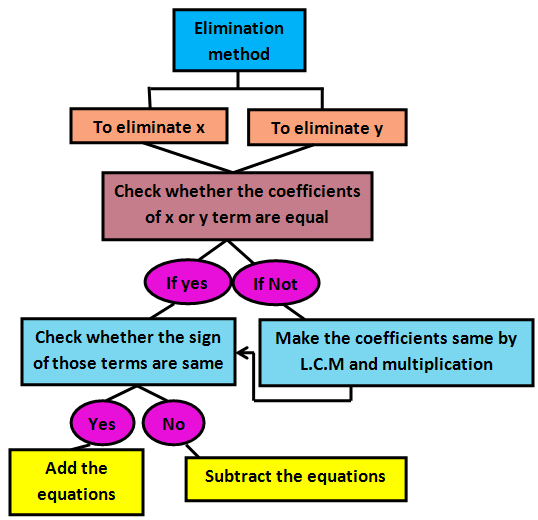Notice: Undefined index: options in /home/u337987201/domains/classnotes.ng/public_html/wp-content/plugins/elementor-pro/modules/theme-builder/widgets/site-logo.php on line 192
Simultaneous Linear Equations In One Or Two Variables - ClassNotes.ng

# Simultaneous Linear Equations In One Or Two Variables

Welcome to JSS3 Second Term!

In today’s class, We will be discussing Simultanoues LInear Equations II. We hope you enjoy the class!SIMULTANEOUS EQUATION

An equation such as 4x + 1=7 has only one solution and one unknown, thus it is called a linear equation. Considering equations such as 4x +2y = 24 which contains two unknown quantities (x, y) it cannot be solved unless one of the variables is given or another connecting the variable is given.  Hence we have two linear such as x+y=10; and x-y=2, this is known as a simultaneous equation. To solve the given equations (simultaneous equation), we need to find the value of x and value of y that will satisfy both equations at the same time.

SUBSTITUTION METHOD OF SOLVING SIMULTANEOUS EQUATION

In using this method, one of the variables is made the subject of the equation. Then the value of the subject of the equation is substituted in the second equation. When the substitution is done, the equation is solved to obtain the value of one of the variables. The value is then substituted in one of the pair of equations to find the second variable.

Example: solve this pair of simultaneous equations using the substitution method;

X+6y = -2

3x+2y =10

Solution:

X+6y = -2………(1)

3x+2y=10 ………(2)

From eq (1) x= -2 -6y

Sub x= -2 -6y in eq(2)

3(-2-6y) +2y = 10

-6-18y +2y =10

-16y =10+6

-16y =16

y=16/-16

y=-1

sub y = -1 in eq(1)

x +6y= -2

x +6(-1) = -2

x-6 = -2

x=-2+6

x = 4

Self Evaluation:

1. x+3y =-4 ; x +y=-10           2. 5x-y=35; 3x-2y=14

ELIMINATION METHOD OF SOLVING SIMULTANEOUS EQUATION

In the Elimination method, the two simultaneous equations are either added or subtracted so as to eliminate one of the variables. This is very useful to solve simultaneous equation especially when none of the coefficients of the unknown is one (1).

Example: use Elimination method to solve; 6x +5y=2 and x-5y=12

Solution:

6x+5y=2

X-5y=12

Hence, x = 2

sub x = 2 in eq(1)

6x+5y =2

6(2)+5y =2

12+5y =2

5y= 2-12;         5y=-10

y= -10/5 ;

Hence, y= -2SELF EVALUATION:  simplify using Elimination method;

1. 2y-x=10; y+x=2      2. 4p+3q;   3p-5q=-10

WORD PROBLEMS LEADING TO SIMULTANEOUS EQUATION

To solve such problems:

1. Identify the two unknowns and represent them with letters.
2. Translate the words into equations.
3. Use any convenient method to solve the two unknowns.

Example:

The difference between the ages of Audu and Ojo is 15. if the sum of their ages is 47. How old are they?

Solution: let x represents Audu’s age and y represent Ojo’s age.

x-y =15…………(1)

x+y =47………..(2)

x= 62/2   =31

Substituting:        x-y=15

31-y=15

-y=15-31

-y=-16

y=16

SELF EVALUATION:

1. The cost of one orange and two apples is 24k. Two orange and three apples cost 44k. How much does each cost?
2. Six pencils and three rubbers cost N117. Five pencils and two rubbers cost N92. How much does each cost?

We have come to the end of this class. We do hope you enjoyed the class?

Should you have any further question, feel free to ask in the comment section below and trust us to respond as soon as possible.

In our next class, we will be talking about Simultaneous Linear Equations II. We are eager to meet you there.

For more class notes, videos, homework help etc download our Mobile App HERE

Join ClassNotes.ng Telegram Community HERE for exclusive content and support

### 4 thoughts on “Simultaneous Linear Equations In One Or Two Variables”

1.Ayodele blessing

So lovely,i enjoyed d teaching,

2.3.4.